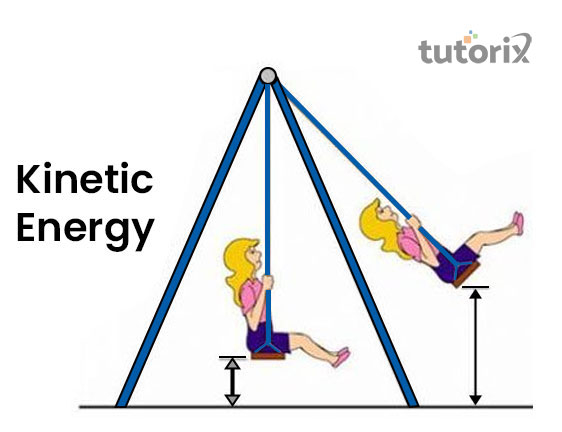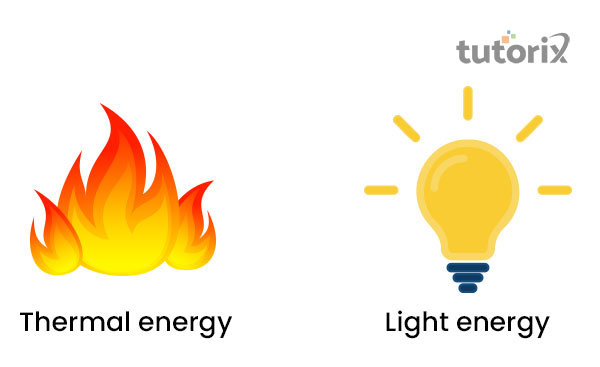# Types of Energy

## Introduction

Energy is abundant in nature and it exists in multitudes of forms that can transform from one form of energy into another. These energies are mainly classified into potential and kinetic energy these energies can however be sub-divided into various other categories that have their own set of distinct functionalities and principles. These energies however can be subdivided into various other forms like gravitational energy, atomic energy and many more.

The kinetic energy is the kind of energy that is mostly available in the moving objects. The potential energy is classified into the form of energy that is stored for future purposes.

## What is energy?

The definition of energy can be described in very layman's terms, the energy is defined as the ability to perform a task or doing a work. Biologically, it can be defined as the ability to make an impact in such a manner that would lead to certain changes. Energy however can be classified into various forms, such as light, heat and electrical energy.

The classification of the energy can be considered using two primary categories that are kinetic energy and potential energy.

The first is associated with the energy of motion while the latter is associated with the storing of energy (eia 2022).

There is however another form of energy, that is ,the chemical energy this energy is about the form of energy that is found in the chemical bonds as the potential energy(ypte 2022). It is to be noted about the energy that it is never lost, however converts from one form into another. It is a scalar quantity and its SI unit is determined in Joule.

## Energy: different forms

There are different forms of energy that can be found in nature. The forms of energy are classified into two key categories, the potential energy and the the kinetic energy. The types of energy will be elaborated further.

• Kinetic energy − This is the form of energy that is termed the energy of motion that is if the object is mobile energy in the body is concluded to have kinetic energy. Along with potential energy, this is one of the primary forms of energy(coredifferences 2022). This energy is experienced by a person on a swing.Figure 1: Kinetic energy

• Potential energy − This energy resides within a particular object due to the arrangement, position or a particular state. The potential energy is quantified by the amount of work that has been done (libretexts 2022). Potential energy is given by m*g*h. Here ‘m’ is the mass of the object it is measured n kilograms, g is the acceleration because of gravity and ‘h’ is the height that is measured in meters.

For example, water in a lake is noted to have potential energy.

• Mechanical energy − The total of potential and kinetic energy that is related to the motion and the position of the object is termed the mechanical energy.
• Chemical energy − This form of energy is mostly stored in the bond of the chemical compounds. In a particular environment and at a distinct temperature the energy can either be released or absorbed because of the chemical reactions. Usually when the chemical energy is released the product transforms into a completely new product.

The chemical energy is mostly available in the chemical batteries, airbags of the vehicles.Figure 2: Thermal and Light energy

• Light energy − This energy is mostly classified as electromagnetic radiation. The light is consistent with photons that are produced by heating atoms. The light travels in waves and is the only form of energy that is visible with naked eyes. This energy is also classified as kinetic energy.
• Thermal energy − The thermal energy is also considered as the heat energy. As opined by Alva et al. (2018)This is produced with the temperature rise makes the atoms and molecules become more agile and eventually collide with one another. The energy thus derived is termed as the thermal energy, example, the heat of the sun, oven baking etc.

## Types of energy resources

The energy source can also be classified into fuels. The fuels thus derived can be used in automobiles and different machinery. These fuels have their set of application in the thermal plants that helps in the generation of electricity. Energy resources are classified into Non-renewable and renewable sources of energy.

• Non-renewable sources of energy are the fossil fuels like natural gas, oil and coal. These energy resources cannot be renewed by natural means.
• Renewable resources are the natural resources that are found in water, wind, solar and geothermal energies. These energies can continuously regenerate and are abundantly available in nature. The geothermal, hydropower, nuclear, bio-energy and solar energy are classified into energy sources based on their resources.

## Conclusion

Energy is found in abundance in different forms and resources. All the energy forms that are to be found are classified into potential or kinetic energy. The potential energy is the energy that is stored for future endeavors and the kinetic energy is found in the objects that are in a locomotive state. There are various types of energy to be found in nature and these energies can be classified into two major section sections that are renewable and non-renewable energy based on their distinct type of resources. The first is associated with the energy that replenishes over time while the non-renewable energy does not regenerate back.

## FAQs

Q1. What is electrical energy?

This energy is obtained from the moving electrons found in the conductor. The natural source of this energy is lightning.

Q2. What is ionization energy?

It is the form of energy that helps in binding electrons with the nucleus of the atoms, ions and molecules. The second ionization energy is greater than the first ionization energy for the removal of electrons.

Q3. What is gravitational energy?

The energy is associated with the attraction of two objects. For example, the gravitational energy binds atmosphere to the earth.

Q4. What is kinetic energy?

Kinetic energy is the energy of bodily motion. A walking person is an example of this energy.